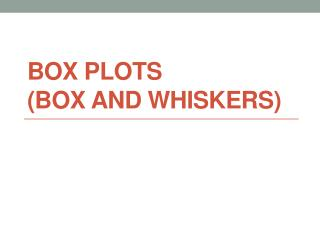DownloadDownload PresentationBox Plots (Box and Whiskers)

# Box Plots (Box and Whiskers)

Télécharger la présentation## Box Plots (Box and Whiskers)

- - - - - - - - - - - - - - - - - - - - - - - - - - - E N D - - - - - - - - - - - - - - - - - - - - - - - - - - -
##### Presentation Transcript

1. Box Plots(Box and Whiskers)

2. Boxplot • A graph of a set of data obtained by drawing a horizontal line from the minimum to maximum values with quartiles labeled in between. • It is a graphical plot of 5 specific values called the 5-number summary.

3. 5-number summary….. Minimum – lowest number in set Q1- middle number of the lower half Median – middle number of entire set Q3 – middle number of upper half Maximum – highest number in set

4. 1. Find the 5-number summary. 2. Draw and label a scale of equal intervals. 3. Place dots above the 5 numbers 4. Put a box around Q1 and Q3. 5. Draw a vertical line through the median. 6. Draw “whiskers” from the minimum to Q1 and maximum to Q3. Steps……

5. Example…… • Draw a box plot of the following data. 33, 38, 43, 30, 29, 40, 51, 27, 42, 23, 31

6. Example…… • Draw a box plot of the following data. 59, 60, 65, 52, 51, 64, 71, 48, 40, 50

7. 5, 8, 13, 17, 22, 24, 25, 27, 29, 30

8. 8, 10, 22, 24, 25, 25, 26, 27, 45, 72 • Graph & Describe

9. 5, 7, 9, 9, 10, 11, 13, 17, 29 • Graph the Boxplot.

10. 22, 27, 33, 39, 57, 88, 110

11. Comparative • Measure your longest finger. • Create boxplot of right vs left. • Describe

12. Assignment…… • Worksheet (Both sides).